# Maximum Power Transfer Theorem Explained with Examples

In any electric and electronic circuit, the power supply is used to provide electrical energy to the load wherever it is changed into functional work. Basically, the whole power supply will not there at load because of the heating effect as well as other restrictions within the circuit. So, there exists a certain difference between drawing and delivering powers. The size of the load will affect the sum of power delivered from the source of power supply, any change within the load resistance can result to modify within power transfer toward the load. So, the maximum power transfer theorem or MPTT ensures the condition to deliver the maximum power toward the load.

In any electronic or electrical applications, the sum of power obtained through a load is an essential parameter. For instance, the load in DC circuits can be represented through a resistor with resistance ‘RL’ ohms. In the same way in AC circuits, we can indicate it through a complex load with impedance ‘ZL’ ohms. This article discusses an overview of MPPT (Maximum Power Transfer Theorem).

## What is the Maximum Power Transfer Theorem?

The Maximum Power Transfer Theorem can be defined as, a resistive load is connected to a DC-network, when the load resistance (RL) is equivalent to the internal resistance then it receives the highest power is known as Thevenin’s equivalent resistance of the source network. The theorem defines how to select the load resistance (RL) when the source resistance is given once. It is a general misunderstanding for applying the theorem in the reverse situation.

It doesn’t mean that how to select the source resistance for a specific load resistance (RL). Actually, the source resistance that makes the best use of power transfer is constantly zero, apart from the value of load resistance. This theorem can be expanded to AC circuits that comprise reactance and defines that the highest power transmission happens when the load impedance (ZL) must be equivalent to the ZTH (complex conjugate of corresponding circuit impedance).

### Maximum Power Transfer Theorem Solved Problems

1). Find the load resistance RL that enables the circuit (left of the terminals a and b) to deliver maximum power toward the load. Also, find the maximum power delivered to the load.

Solution:

In order to apply the maximum power transfer theorem, we need to find Thevenin’s equivalent circuit.

(a) Vth derivation of the circuit: open-circuit voltage

Constraints: V1=100, V2 – 20=Vx, and V3=Vth

At node 2: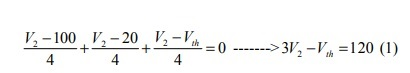At node 3: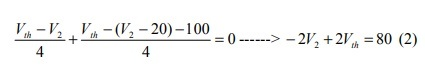(1)*2 + (2)*3 –> Vth=120 [V]

(b) Rth derivation (by Test Voltage Method): After deactivation & test voltage application, we have:

Constraints: V3=VT and V2=Vx

At node 2: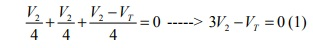At node 3 (KCL):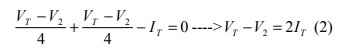From (1) and (2):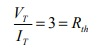(c) Maximum Power Transfer: now the circuit is reduced to:

To obtain maximum power transfer, then, RL=3=Rth. Finally, the maximum power transferred to RL is: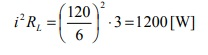2). Determine the maximum power that can be delivered to the variable resistor R.

Solution:

(a) Vth: Open circuit voltage

From the circuit, Vab=Vth=40-10=30 [V]

(b) Rth: Let’s apply Input Resistance Method:

Then Rab= (10//20) + (25//5) = 6.67+4.16=10.83 =Rth.

(c) Thevenin circuit: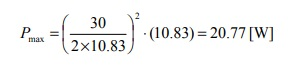### MPTT Formula

The Maximum Power Transfer Theorem Formula is explained below. If we consider the η (efficiency) as the fraction of power dissolved through the load R to power extended with the source, VTH, then it is simple to compute the efficiency of maximum power transfer as

η = (Pmax/P) X 100 = 50%

Where; the maximum power (Pmax)

Pmax = V2TH RTH / (RTH + RTH)2 = V2TH / 4RTH

And the power supplied (P) is

P = 2 V2TH / 4RTH = V2TH / 2RTH

The η is only 50% when the highest power transfer is attained, although reaches 100% as the RL (load resistance) reaches infinity, while the whole power stage tends to zero.

#### Maximum Power Transfer Theorem for AC Circuits

In an AC circuit, the MPTT provides the conditions of impedance to transfer the highest power toward a load. This theorem states that in an active AC circuit, a source through internal impedance like ZS is included which is connected to a load like ZL. So, the maximum power transfer mainly happens from source to load once the load impedance is equivalent to the compound conjugate of ZS or source impedance.

As inactive arrangement, the highest power is transmitted to the load while the impedance of the load is equivalent to the complex conjugate of a corresponding impedance of a given set-up as observed from the terminals of the load.

The above circuit is an equivalent circuit to Thevenin’s. When the above circuit is considered across the terminals of the load, then the flow of current will be given as

I = VTH / ZTH + ZL

Where ZL = RL + jXL

ZTH = RTH + jXTH

Therefore,

I = VTH / (RL + jXL + RTH + jXTH )

= VTH / ((RL+ RTH) + j(XL + XTH ))

The power circulated to the load,

PL = I2 RL

PL = V2TH × RL / ((RL+ RTH)2 + (XL + XTH )2) ……(1)

For highest power the above equation derivative should be zero, later than simplification we can get the following.

XL + XTH = 0

XL = – XTH

Substitute the XL value in the above equation 1, and then we can get the following.

PL = V2TH × RL / ((RL+ RTH) 2

Again for the highest power transfer, the above equation derivation must be equivalent to zero, after solving this we can get

RL+ RTH = 2 RL

RL = RTH

Therefore, the highest power will be transmitted from the source to load, if RL (load resistor) = RTH & XL = – XTH in an AC circuit. This means that the load impedance (ZL) must be equivalent to the ZTH (complex conjugate of corresponding circuit impedance)

ZL = ZTH

This maximum power transmitted (Pmax) = V2TH/ 4 RL or V2TH / 4 RTH

#### Maximum Power Transfer Theorem for DC Circuits

The MPTT is used in DC circuits which defines the statement of highest power transfer from an active network toward an exterior load resistance. It defines that in an active, linear, bilateral DC n/w, the highest power will be delivered from the power source to the electric load once the exterior load resistance equivalents to the power source’s internal resistance.

The development of MPTT can be done through reference to a voltage source or practical current. If the power source is an independent voltage source or practical, then its inside series resistance should equal load resistance to transmit the highest power.

An independent current source or practical, parallel internal resistance must be equal by the load resistance. The internal source series resistance in the DC circuit changes the power transmitted to the load and thus the highest current transmitted from the power source toward the electric load is restricted.

### MPTT Proof

The maximum power transfer theorem-proof is, in some applications, the purpose of a circuit is to provide maximum power to a load. Some examples:

• Stereo amplifiers
• Communications equipment

If the entire circuit is replaced by its Thevenin equivalent circuit, except the load, as shown below, the power absorbed by the load is:

PL = i2 RL = (Vth/Rth + RL)2 x RL = V2th RL/ (Rth + RL)2

As VTH and RTH are fixed for a given circuit, the load power is a function of the load resistance RL.

By differentiating PL with respect to RL and set the result equal to zero, we have the following maximum power transfer theorem; Maximum power occurs when RL is equal to RTH.

When the maximum power transfer condition is met, i.e., RL=RTH, the maximum power transferred is:

PL = V2th RL / [Rth + RL]2 = V2th Rth/ [Rth + RL]2 = V2th/ 4 Rth

### Steps for Solving Network using Maximum Power Transfer Theorem

Below steps are used to solve the problem by Maximum Power Transfer Theorem

Step 1: Remove the load resistance of the circuit.

Step 2: Find the Thevenin’s resistance (RTH) of the source network looking through the open-circuited load terminals.

Step 3: As per the maximum power transfer theorem, RTH is the load resistance of the network, i.e., RL = RTH that allows maximum power transfer.

Step 4: Maximum Power Transfer is calculated by the below equation

(Pmax) = V2TH / 4 RTH

#### Maximum Power Transfer Theorem Example Problems with Solutions

Find the RL value for the below circuit that the power is highest as well, find the highest power through RL using the theorem of maximum power transfer.

Solution:

According to this theorem, when power is highest via the load, then resistance is similar to the equal resistance between the two ends of the RL after eliminating it.

So, for load resistance (RL) discovery, we have to discover the equivalent resistance: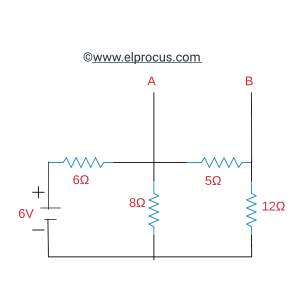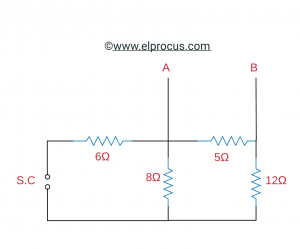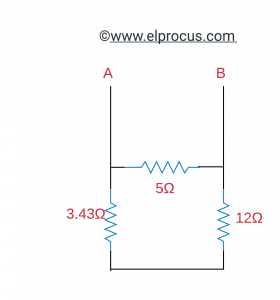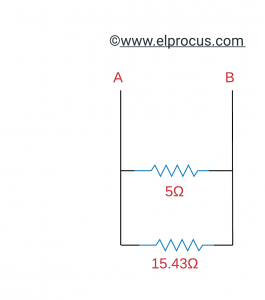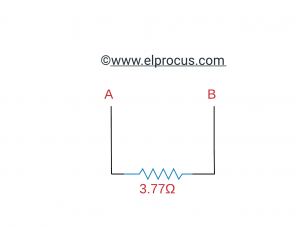So,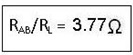Now, for discovering the highest power through RL-load resistance, we have to discover the voltage value between the VOC circuits.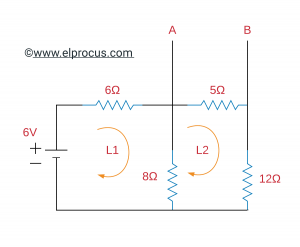For the above circuit, apply the mesh analysis. We can get:

Apply KVL for loop-1:

6-6I1-8I1+8I2=0

-14I1+8I2=-6         ∙∙∙∙∙∙∙∙∙∙∙∙∙∙∙∙∙∙∙∙∙∙∙∙∙∙∙∙∙∙∙∙ (1)

Apply KVL for loop-2:

-8I2-5I2-12I2+8I1=0

8I1-25I2=0             ∙∙∙∙∙∙∙∙∙∙∙∙∙∙∙∙∙∙∙∙∙∙∙∙∙∙∙∙∙∙∙∙ (2)

By solving the above two equations, we get

I1 = 0.524 A

I2 = 0.167 A

Now, from the circuit Vo.c is

VA-5I2- VB = 0

Vo.c/ VAB= 5I2 = 5X0.167 = 0.835v

Hence, the maximum power through the load resistance (RL) is;

P max = VOC2 / 4RL = (0.835 x 0.835)/ 4 x 3.77 = 0.046 watt

Discover the highest power that can be transmitted to the RL-load resistor of the below circuit.

Solution

Apply Thevenin’s theorem to the above circuit,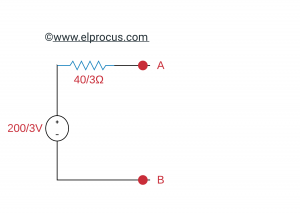Here, Thevenin’s voltage (Vth)= (200/3) and Thevenin’s resistance (Rth)=(40/3)Ω

Substitute the fraction of the circuit, which is left-side of terminals A & B of the given circuit with the Thevenin’s equivalent circuit. The secondary circuit diagram is shown below.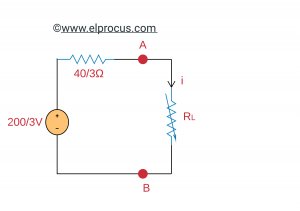We can find the maximum power that will be delivered to the load resistor, RL by using the following formula.

PL, Max = V2TH / 4 RTH

Substitute VTh = (200/3)V and RTh = (40/3)Ω in the above formula.

PL, Max = (200/3)2/ 4(40/3) = 250/3 watts

Therefore, the maximum power that will be delivered to the load resistor RL of the given circuit is 250/3 W.

The advantages and disadvantages of the maximum power transfer theorem include the following.

• The main benefit of the maximum power transfer theorem is, it can analyze the performance at any point
• The main drawback of the maximum power transfer theorem is, it is not used in the networks like nonlinear as well as unilateral.
• The limitation of the maximum power transfer theorem is it not applicable in power systems, due to its 50% efficiency. So the main concern of this is efficiency.
• This theorem can be applied to communication lines instead of power lines because if we applied to power lines, then practical problems will occur like the following.
• In power lines, receiving end voltage constancy is a significant condition, so this theorem ignores this feature.
• Due to less efficiency, this cannot be accepted within power lines.

### Applications of Maximum Power Transfer Theorem

The theorem of maximum power transfer can be applicable in many ways to determine the load resistance’s value that receives the maximum power from the supply and the maximum power under the state of the highest power transfer. Below are a few applications of the Maximum power transfer theorem:

• This theorem is always sought in a communication system. For instance, in a community address system, the circuit is attuned for the highest power transfer with making the speaker (load resistance) equivalent to the amplifier (source resistance). When the load and source have matched then it has equal resistance.
• In automobile engines, the power transmitted to the motor starter of the automobile will depend on the effective resistance of the motor & the battery’s inner resistance. When the two resistances are equivalent, then the highest power will be transmitted to the motor to activate the engine.
• The maximum power transfer theorem can be applied in the complex network as follows
• It is used in ac and dc networks.
• For maximum power transfer in the public address system, the circuit can be altered by making RL (load resistance) like speaker equivalent toward the source resistance like an amplifier. Once both the source as well as load includes the equivalent resistance, then they are matched.
• This theorem is used wherever all the autonomous voltage sources are deactivated & the same impedance is simply considered, then a maximum quantity of power can be transmitted.
• In radio communications, it is used where the power amplifier broadcasts the highest amount of signal toward the antenna if load impedance within the circuit is equivalent to the impedance of the source.
• In audio systems, it is used where the need to be delivered toward the speaker. The amplifier changes the highest quantity of voice once the load impedance is equivalent to the source impedance.
• In a resistive network, a resistive load will abstract maximum power once the load resistance is equivalent to the resistance observed through the load because it looks back toward the network.
In fact, the resistance can be accessed toward the network output terminals. Actually, this resistance is Thevenin equivalent resistance which is described in Thevenin’s theorem if we consider the complete network like a voltage source. Likewise, if we consider the network like a current source, then this resistance will be called Norton equivalent resistance which can be described in the Norton theorem.
• It is used in all kinds of circuits where the circuits are designed based on the efficiency principle which will reduce by 50%. However, consumers use this MPTT in areas wherever power is restricted such as mobile receivers & audio speakers.
• This less efficiency can be bearable for the sake of the highest power to be delivered toward the load used in the network. In power systems, the voltage drops & power losses within the generators, as well as the transmission systems, are set aside like small.
• Here, the load impedance is higher several times as compared to the internal impedance of the source
• Therefore, power circuits work below the conditions of optimal matching. For instance, in power transmission wherever the highest efficiency matters, so we design systems based on the highest voltage. But it is not possible to resist heat dissipation because of 50% loss, as the power delivered is a hundred MW.

This is all about the maximum power theorem. From the above information, finally, we can conclude that this theorem is used often to assure that the highest power can be transmitted from a source of power to a load. Here is a question for you, what is the advantage of the maximum power transfer theorem?